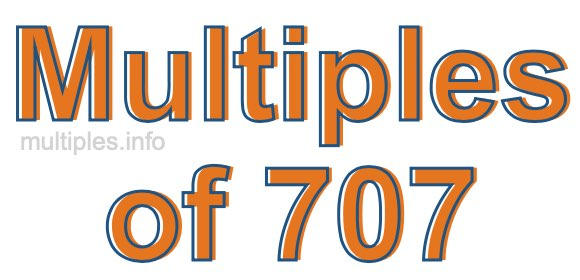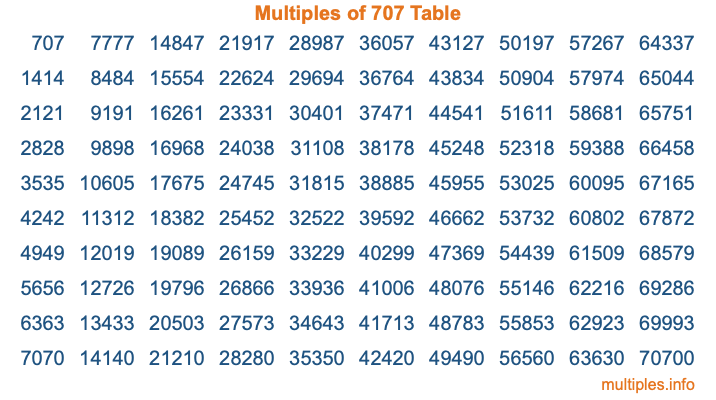Multiples of 707Welcome to the Multiples of 707 page. Here we will first teach you everything you will ever need to know about the multiples of 707, and then give you a study guide summary of everything we taught you to make sure you remember it all. Use this page to look up facts and learn information about the multiples of 707. This page will make you a multiples of seven hundred seven expert!

Definition of Multiples of 707
Multiples of 707 are all the numbers that when divided by 707 equal an integer. Each of the multiples of 707 are called a multiple. A multiple of 707 is created by multiplying 707 by an integer.

Therefore, to create a list of multiples of 707, you start with 1 multiplied by 707, then 2 multiplied by 707, then 3 multiplied by 707, and so on for as long as you want. Thus, the list of the first five multiples of 707 is 707, 1414, 2121, 2828, and 3535. To see a larger list of multiples of 707, see the printable image of Multiples of 707 further down on this page. We also have a category where you can choose any nth multiple of 707.

Multiples of 707 Checker
The Multiples of 707 Checker below checks to see if any number of your choice is a multiple of 707. In other words, it checks to see if there is any number (integer) that when multiplied by 707 will equal your number. To do that, we divide your number by 707. If the the quotient is an integer, then your number is a multiple of 707.

Is  a multiple of 707?

Least Common Multiple of 707 and ...
A Least Common Multiple (LCM) is the lowest multiple that two or more numbers have in common. This is also called the smallest common multiple or lowest common multiple and is useful to know when you are adding our subtracting fractions. Enter one or more numbers below (707 is already entered) to find the LCM.

Check out our LCM Calculator if you need more details about the Least Common Multiple or if you need the LCM for different numbers for adding and subtraction fractions.

nth Multiple of 707
As we stated above, 707 is the first multiple of 707, 1414 is the second multiple of 707, 2121 is the third multiple of 707, and so on. Enter a number below to find the nth multiple of 707.

th multiple of 707

Multiples of 707 vs Factors of 707
707 is a multiple of 707 and a factor of 707, but that is where the similarities end. All postive multiples of 707 are 707 or greater than 707. All positive factors of 707 are 707 or less than 707.

Below is the beginning list of multiples of 707 and the factors of 707 so you can compare:

Multiples of 707: 707, 1414, 2121, 2828, 3535, etc.

Factors of 707: 1, 7, 101, 707

As you can see, the multiples of 707 are all the numbers that you can divide by 707 to get a whole number. The factors of 707, on the other hand, are all the whole numbers that you can multiply by another whole number to get 707.

It's also interesting to note that if a number (x) is a factor of 707, then 707 will also be a multiple of that number (x).

Multiples of 707 vs Divisors of 707
The divisors of 707 are all the integers that 707 can be divided by evenly. Below is a list of the divisors of 707.

Divisors of 707: 1, 7, 101, 707

The interesting thing to note here is that if you take any multiple of 707 and divide it by a divisor of 707, you will see that the quotient is an integer.

Multiples of 707 Table
Below is an image of the first 100 multiples of 707 in a table. The table is in chronological order, column by column. The first column has the first ten multiples of 707, the second column has the next ten multiples of 707, and so on.The Multiples of 707 Table is also referred to as the 707 Times Table or Times Table of 707. You are welcome to print out our table for your studies.

Negative Multiples of 707
Although not often discussed or needed in math, it is worth mentioning that you can make a list of negative multiples of 707 by multiplying 707 by -1, then by -2, then by -3, and so on, to get the following list of negative multiples of 707:

-707, -1414, -2121, -2828, -3535, etc.

Multiples of 707 Summary
Below is a summary of important Multiples of 707 facts that we have discussed on this page. To retain the knowledge on this page, we recommend that you read through the summary and explain to yourself or a study partner why they hold true.

There are an infinite number of multiples of 707.

A multiple of 707 divided by 707 will equal a whole number.

707 divided by a factor of 707 equals a divisor of 707.

The nth multiple of 707 is n times 707.

The largest factor of 707 is equal to the first positive multiple of 707.

707 is a multiple of every factor of 707.

707 is a multiple of 707.

A multiple of 707 divided by a divisor of 707 equals an integer.

707 divided by a divisor of 707 equals a factor of 707.

Any integer times 707 will equal a multiple of 707.

Multiples of a Number
Here you can get the multiples of another number, all with the same attention to detail as we did for multiples of 707 on this page.

Multiples of
Multiples of 708
Did you find our page about multiples of seven hundred seven educational? Do you want more knowledge? Check out the multiples of the next number on our list!